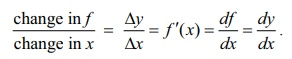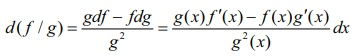Home | | Maths 12th Std | Differentials

# Differentials

Here again, we use the derivative concept to introduce ‚ÄúDifferential‚Äù.

Differentials

Here again, we use the derivative concept to introduce ‚ÄúDifferential‚Äù. Let us take another look at (1),Here df/dx is a notation, used by Leibniz, for the limit of the difference quotient, which is called the differential coefficient of y with respect to x .Will it be meaningful to treat df/dx as a quotient of df and dx ? In other words, is it possible to assign meaning to df and dx so that derivative is equal to the quotient of df and dx .Well, in some cases yes. For instance, if f ( x) = mx + c , m, c are constants, then, y = f ( x) .

Œîy = f ( x + Œîx ) ‚àí f (x) = mŒîx = f ‚Ä≤(x)Œîx for all x ‚àà ‚Ñù and Œîx

and hence equality in both (2), and (3). In this case changes in x and y (= f ) are taking place along straight lines, in which case we have,

change in f / change in x =Thus in this case the derivative df/dx is truly a quotient of df and dx, if we take df = Œîf = dy and dx = Œîx. This leads us to define the differential of f as follows:

### Definition 8.4

Let f : (a, b) ‚Üí ‚Ñù be a differentiable function, for x ‚àà(a, b) and Œîx the increment given to x , we define the differential of f by

df = f ‚Ä≤ (x)Œîx . ... (8)

First we note that if f (x) = x , then by (8) we get dx = f ‚Ä≤(x)Œî x = 1Œîx which means that the differential dx = Œîx , which is the change in x -axis. So the differential given by (8) is same as df = f ‚Ä≤(x)dx .Linear Approximation and Differential

Next we explore the differential for an arbitrary differentiable function y = f (x).

Then Œîf = f ( x + dx ) ‚àí f (x) gives the change in output along the graph of y = f (x) and f ‚Ä≤(x) gives the slope of the tangent line at ( x , f (x)) . Let dy or df denote the increment in f along the tangent line. Then by the above observation, we have dy = f ‚Ä≤(x)dx .

From the figure it is clear that Œîf ‚âà dy = df = f ‚Ä≤(x)dx and hence f ‚Ä≤ (x) can be viewed approximately as the quotient of Œîf and Œîx. So we may interpret df/dx as the quotient of df and dx .

### Remark

We know that derivative of a function is again a function. On the other hand, differential df of a function f is not only a function of the independent variable but also depends on the change in the input namely dx = Œîx . So df is a function of two changing quantities namely x and dx. Observe that Œîf‚âà df , which can be observed from the Fig. 8.4.

In the table below we give some functions, their derivatives and their differentials side by side for comparative purpose.Next we look at the properties of differentials. These results easily follow from the definition of differential and the rules for differentiation. We give a proof for (5) below and the other proofs are left as exercises.

## Properties of Differentials

Here we consider real-valued functions of real variable.

(1) If f is a constant function, then df = 0 .

(2) If f (x) = x identity function, then df = 1dx .

(3) If f is differentiable and c ‚àà R, then d(cf) = cf ‚Ä≤(x)dx .

(4) If f , g are differentiable, then d ( f + g) = df + dg = f ‚Ä≤ (x)dx + g‚Ä≤(x)dx .

(5) If f , g are differentiable, then d ( fg ) = fdg + gdf = ( f (x) g ‚Ä≤ ( x) + f ‚Ä≤ (x) g ( x))dx.

(6) If f , g are differentiable, then, where g(x)‚âÝ0.

(7) If f , g are differentiable and h = f o g is defined, then dh = f ‚Ä≤( g( x )) g‚Ä≤( x ) dx .

(8) If h(x) = ef (x) , then dh = ef (x) f ‚Ä≤( x ) dx .

(9) If f (x) > 0 for all x and g (x) = log( f (x)) , then dg = f ‚Ä≤(x)/f(x) dx .

### Example 8.5

Let f , g : (a , b) ‚Üí ‚Ñù be differentiable functions. Show that d(fg ) = fdg + gdf .

### Solution

Let f , g : (a , b) ‚Üí ‚Ñù be differentiable functions and h(x) = f (x) g (x) . Then h , being a product of differentiable functions, is differentiable on (a, b) . So by definition dh = h‚Ä≤ ( x)dx. Now by using product rule we have h‚Ä≤ (x) = f (x) g‚Ä≤ ( x) + f ‚Ä≤(x) g(x) .

Thus dh = h‚Ä≤ (x)dx = ( f (x) g‚Ä≤ ( x) + f ‚Ä≤ (x) g( x))dx = f (x) g‚Ä≤ ( x)dx + f ‚Ä≤ (x) g( x)dx

= f (x)dg + g ( x)df = fdg + gdf

Example 8.6

Let g ( x) = x2 + sin x . Calculate the differential dg .

Solution

Note that g is differentiable and g ‚Ä≤(x) = 2x + cos x .

Thus dg = (2x + cos x)dx .

Example 8.7

If the radius of a sphere, with radius 10 cm, has to decrease by 0.1 cm, approximately how much will its volume decrease?

Solution

We know that volume of a sphere is given by V = 4/3 œÄr3 , where r > 0 is the radius. So the differential dV = 4œÄr2dr and hence

ŒîV ‚âà dV = 4œÄ(10)2 (9.9-10) cm3

= 4102 ( 0.1) cm3

= ‚àí 40œÄ cm3.

Note that we have used dr = (9.9 ‚àí10) cm, because radius decreases from 10 to 9.9. Again the negative sign in the answer indicates that the volume of the sphere decreases about 40œÄ cm3.

Tags : Mathematics , 12th Maths : UNIT 8 : Differentials and Partial Derivatives
Study Material, Lecturing Notes, Assignment, Reference, Wiki description explanation, brief detail
12th Maths : UNIT 8 : Differentials and Partial Derivatives : Differentials | Mathematics